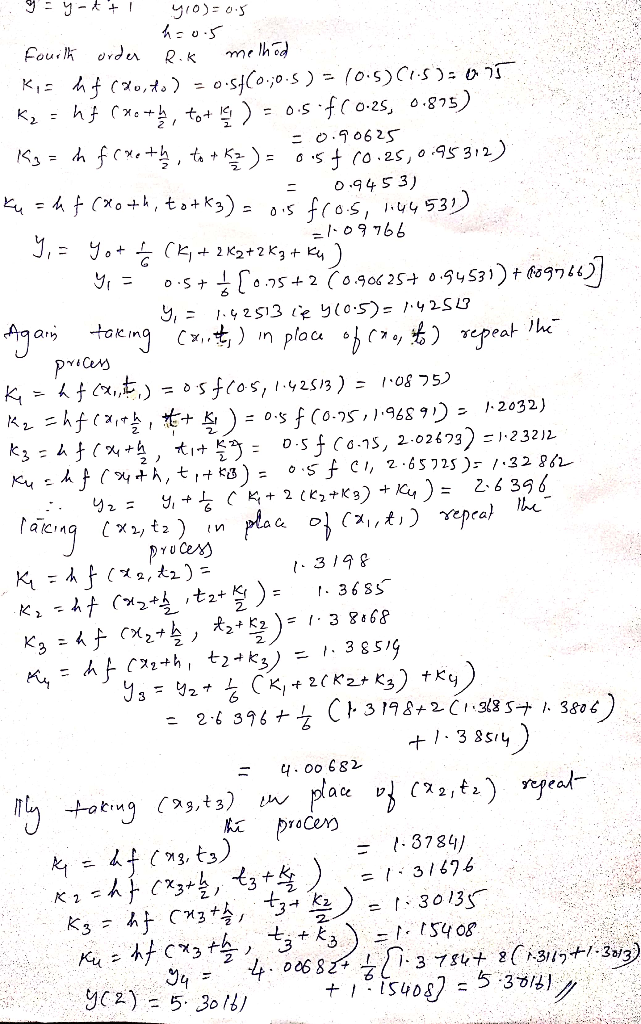# QUESTION 6 (20 Marks) CO CO6 Marks20 Use five decimals points throughout your calculations when solving this question....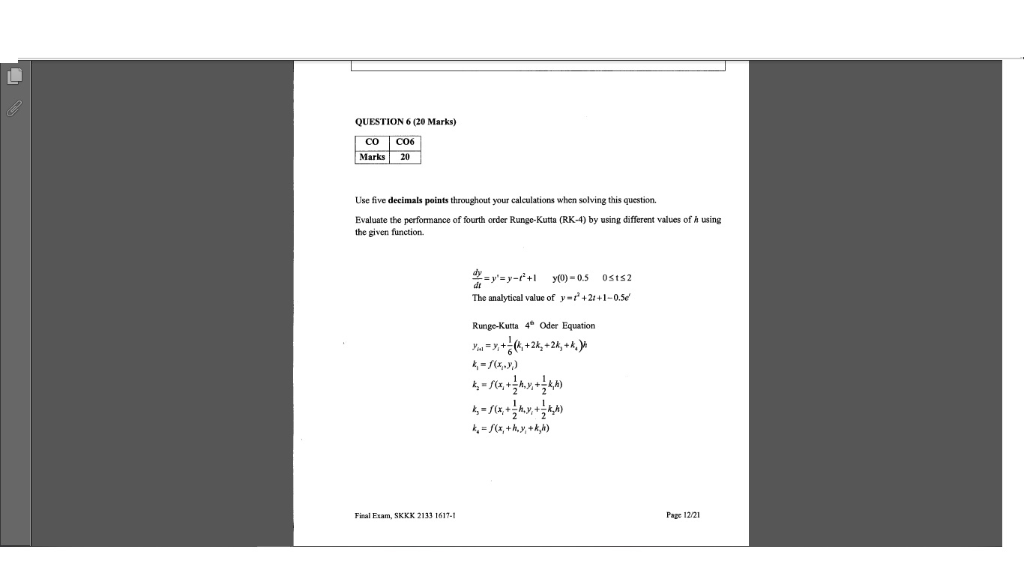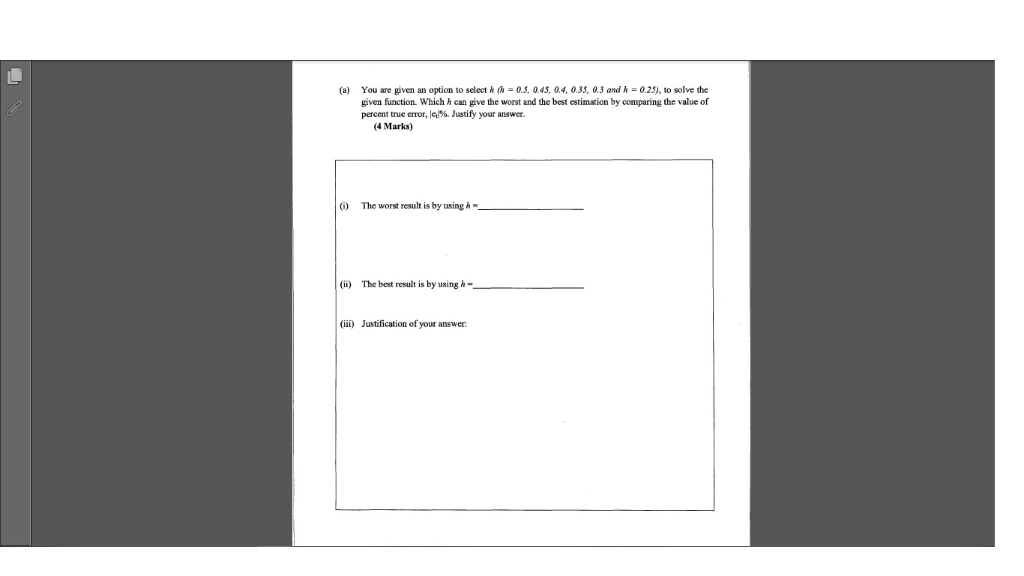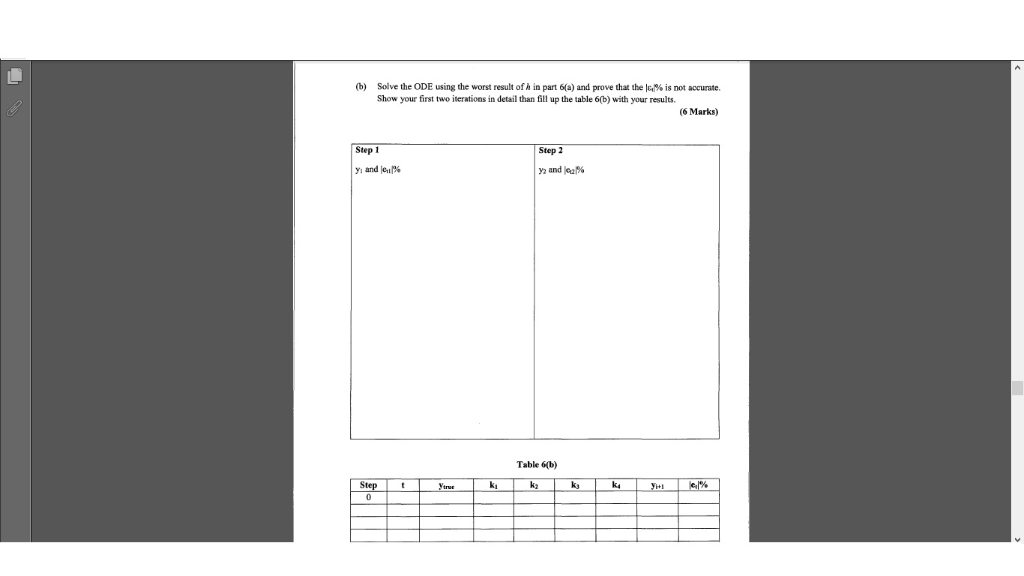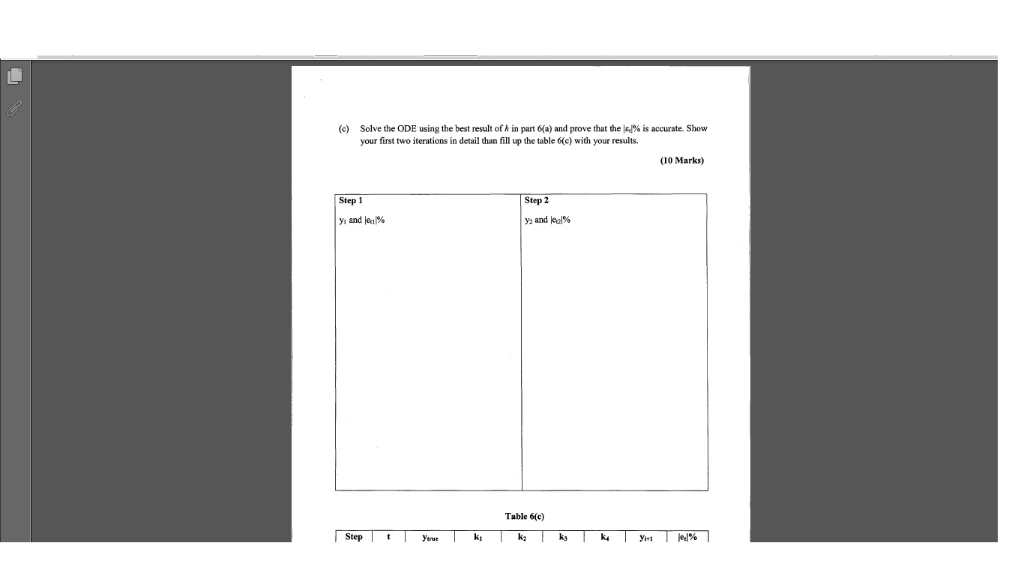QUESTION 6 (20 Marks) CO CO6 Marks20 Use five decimals points throughout your calculations when solving this question. Evaluate the performance of fourth order Runge-Kutta (RK-4) by using different values of h using the given function. The analytical value of y-r+21+1-0.5e Rungo-Kutta 4 Oder Equation Page 12/21 Firal Esam, SKKK 2133 1617-1
You are given an option to select h癰 0.5. a 45, 0.4, 0.35, 0.3 and h 0.25), to solve the (a) given function. Which h can give the worst and the best estimation by comparing the value of percent true cror, lem. Justify your answer. (4 Marks) The worst result is by using h- (ii) The best result is by using h- (ii) Justification of your answer.
(b) Solve the ODE using the worst result of in part 6(a) and prove that the la% is not accurate. Show your first two iterations in detail than fill up the table 6(b) with your results. (6 Marks) Step 1 Step 2 y, and eine Table 6(b) kı ky
(c) Solve the ODE using the best result oFA in part 6(a) and prove that the le)% is accurate. Show your first two iterations in detail than fill up the table 6(e) with your results, (10 Marks) Step 1 Step 2 y, and hed% y, and ki% Table 6(c) Step ki yerue